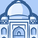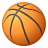# QlikView App Dev

Discussion Board for collaboration related to QlikView App Development.

Announcements
Action-Packed Learning Awaits! QlikWorld 2023. April 17 - 20 in Las Vegas: REGISTER NOW
cancel
Showing results for
Did you mean:Creator III

## 2 set analysis in 1 expression, but only the 1st one works

Hello

I have an expression, which uses 2 set analsysis within it

Count({<data_source_group = State_1 :: data_source_group>} {\$<FactDateNum = {">=\$(=v_Start_Date_ED)<=\$(=v_End_Date_ED)"}>} ED_ID)

However if I place the {<data_source_group = State_1 :: data_source_group>} first, then this part interacts, and if I place {\$<FactDateNum = {">=\$(=v_Start_Date_ED)<=\$(=v_End_Date_ED)"}>}  in the expression first, then this interacts, but I cannot get the 2 too work together

Kind Regards

Helen

1 Solution

Accepted SolutionsMVP

Try like:

Count({<data_source_group = State_1 :: data_source_group , FactDateNum = {">=\$(=v_Start_Date_ED)<=\$(=v_End_Date_ED)"}>} ED_ID)

3 RepliesSpecialist

Try this and let me know

Count(\${<data_source_group = State_1 :: data_source_group,FactDateNum = {">=\$(=v_Start_Date_ED)<=\$(=v_End_Date_ED)"}>} ED_ID)MVP

Try like:

Count({<data_source_group = State_1 :: data_source_group , FactDateNum = {">=\$(=v_Start_Date_ED)<=\$(=v_End_Date_ED)"}>} ED_ID)Creator III
Author

Hello

I moved the \$ sign to inside the set analysis, and the expression parsed, however it did not interact fully with my master calender

The alternative answer which interacts well with my matser calender is:

Count({<data_source_group = State_1 :: data_source_group , FactDateNum = {">=\$(=v_Start_Date_ED)<=\$(=v_End_Date_ED)"}>} ED_ID)

Thanks very much for your help

Thanks

HelenCommunity Browser